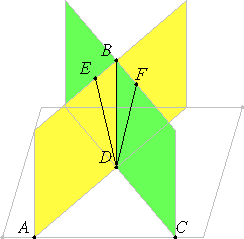# Proposition 19

If two planes which cut one another are at right angles to any plane, then their intersection is also at right angles to the same plane.

Let the two planes AB and BC be at right angles to the plane of reference, and let BC be their intersection.

I say that BD is at right angles to the plane of reference.I.11

Suppose it is not. From the point D draw DE at right angles to the straight line AD in the plane AB, and draw DF at right angles to CD in the plane BC.

XI.Def.4

Now, since the plane AB is at right angles to the plane of reference, and DE is at right angles in the plane AB to AD, their intersection, therefore DE is at right angles to the plane of reference.

XI.13

Similarly we can prove that DF is also at right angles to the plane of reference. Therefore from the same point D two straight lines have been set up at right angles to the plane of reference on the same side, which is impossible.

Therefore no straight line except the intersection DB of the planes AB and BC can be set up from the point D at right angles to the plane of reference.

Therefore, if two planes which cut one another are at right angles to any plane, then their intersection is also at right angles to the same plane.

Q.E.D.

## Guide

This proposition is not used in the rest of the Elements.